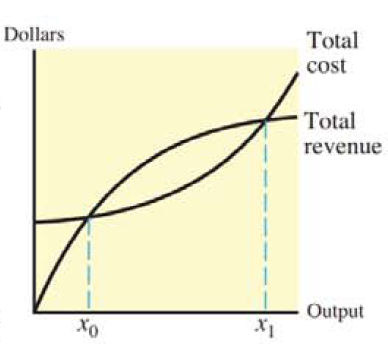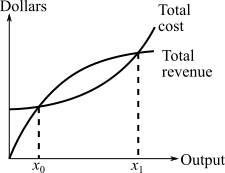Chapter 13.3, Problem 35E### Mathematical Applications for the ...

11th Edition
Ronald J. Harshbarger + 1 other
ISBN: 9781305108042

#### Solutions

Chapter
Section### Mathematical Applications for the ...

11th Edition
Ronald J. Harshbarger + 1 other
ISBN: 9781305108042
Textbook Problem

# Average profit For the product whose total cost and total revenue are shown in the figure, represent total revenue by R(x) and total cost by C(x) and write an integral that gives the average profit for the product over the interval from x 0 to  x 1 .To determine

To calculate: The integral that represents the average profit for the product whose total cost C(x) and total revenue R(x) are shown in the figure over the interval from x0 to x1.Explanation

Given information:

The provided figure is:

Formula used:

Average value

The average value of a continuous function y=f(x) over the interval [a,b] is:

Average value=1baabf(x)dx

Calculation:

The Profit is given by the difference of the Revenue R(x) and the cost C(x) as:

P=R(x)C(x)

Since, R(x) and C(x) are continuous function so is there difference that is Profit so the average value of the Profit in the interval [x0,x1] can be calculated by considering the formula:

Average value=1baabf(x)dx

Substitute x0 for a and x1

### Still sussing out bartleby?

Check out a sample textbook solution.

See a sample solution

#### The Solution to Your Study Problems

Bartleby provides explanations to thousands of textbook problems written by our experts, many with advanced degrees!

Get Started

#### Find more solutions based on key concepts